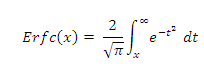Engineering functions

Excel Function CONVERT

The CONVERT() function is used to convert a number from one metric system to another.

The various metric system supported by the function are as follows:

Gram, Slug, Pound, Ounce, Grain,Stone,Ton

Meter, Nautical Mile, Inch, Foot, Yard, Angstrom, Lightyear

Year,Day,Month,Hour,Minute,Second

and various other metrics.

 Number CONVERT Remarks Formula 23 5.07063203025218E-02 Gram to Pound =CONVERT(L5,"g","lbm") 11 12.0297462817148 Meter to Yard =CONVERT(L6,"m","yd") 56 0.00175 Ounce to Ton =CONVERT(L7,"ozm","ton") 97 5820 Hour to Minute =CONVERT(L8,"hr","mn") 5 -268.15 Kelvin to Celcius =CONVERT(L9,"K","C") 14 8.69919669132268E-03 meter/hr to miles/hr =CONVERT(L10,"m/hr","mph") 37 0.049617817315016 Watts to Horsepower =CONVERT(L11,"W","HP")

Excel Function DELTA

The DELTA() function is used to compare two numbers for equality.

This function takes two arguments only.

The function returns 1 if both the numbers are equal and o otherwise.

 Number1 Number2 DELTA Formula 23 23 1 =DELTA(L20,M20) 11 -11 0 =DELTA(L21,M21) 56 22 0 =DELTA(L22,M22) 97 97 1 =DELTA(L23,M23) 5 4 0 =DELTA(L24,M24) 14 14 1 =DELTA(L25,M25) 37 45 0 =DELTA(L26,M26)

Excel Function ERF

The ERF() returns the error function that is integrated between a lower limit and the upper limit.

This function takes two arguments; lower limit and upper limit, both are positive numbers.

Prior to Excel 2010, a negative number on either parameter will result in an error, which has now been fixed.

 Lower limit Upper limit ERF Formula 2 3 4.65564448404865E-03 =ERF(L34,M34) 4 7 1.54172579147627E-08 =ERF(L35,M35) 6 12 0 =ERF(L36,M36) 4 6 1.54172579147627E-08 =ERF(L37,M37) 8 6 0 =ERF(L38,M38) 1 -1 -1.68540158589943 =ERF(L39,M39) 21 21 0 =ERF(L40,M40)

Excel Function ERFC

The ERFC() function returns the complementary of the error function between a lower and infinity.

This function is similar to the ERF except that, this function complements the end value.

The formula for calculating ERFC is as follows:Lower limit ERFC Formula 2 4.67773498104726E-03 =ERFC(L48) 4 1.541725790028E-08 =ERFC(L49) 6 2.15197367124989E-17 =ERFC(L50) 4 1.541725790028E-08 =ERFC(L51) 8 1.12242971729829E-29 =ERFC(L52) 1 0.157299207050285 =ERFC(L53) 21 8.03245387102246E-194 =ERFC(L54)

Excel Function GESTEP

The GESTEP() function checks if the specified number is greater than the step size specified.

This function returns 1 if greater and 0 otherwise.

This function takes two arguments; number and step size.

The step size argument is optional and will taken as 0 if omitted.

 Number Step size GESTEP Formula 2 3 0 =GESTEP(L65,M65) 4 7 0 =GESTEP(L66,M66) 6 12 0 =GESTEP(L67,M67) 4 6 0 =GESTEP(L68,M68) 8 1 =GESTEP(L69,M69) 1 -1 1 =GESTEP(L70,M70) 21 21 1 =GESTEP(L71,M71)